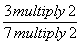## Definition Of Equivalent Ratios

If two ratios have the same value when simplified, then they are called Equivalent Ratios.

Equivalent ratios can be obtained by multiplying or dividing both sides by the same non-zero number.

### Example of Equivalent Ratios

1/3 and 2/6 are equivalent ratios since they represent the same fraction.
The two ratios 8 : 24 and 4 : 12 are equivalent.
There are 10 dolls for every 40 children in a preschool. Then the ratio of the number of children to that of the dolls = 40:10 = 4:1

### Solved Example on Equivalent Ratios

#### Ques: A carnival game has 3 winners for every 7 losers. Find two ratios equal to the ratio of winners to losers.

##### Choices:

A.14/6, 21/9
B.6/14, 10/21
C.7/14, 9/21
D.6/14, 9/21
Step 2: 3/7==6/14 [Multiply both the numerator and the denominator by 2.]
Step 3: 3/7 == 9/21[Multiply both the numerator and the denominator by 3.]